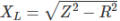# what is the inductance L for this coil?

Joined Jul 18, 2013
28,050
Do you know the application it was used in?
Looks like mains, or other low freq. choke 50/60hz DC supply filter..

#### Ian0

Joined Aug 7, 2020
8,947
Do you have a variac? If so, it's easy to measure.

#### idir93

Joined Jan 10, 2018
27
It is used in a vibratory feeder. frequency is controlled by REOVIB MFS 268.

#### idir93

Joined Jan 10, 2018
27
Do you have a variac? If so, it's easy to measure.
Yes I had the idea actually. XL=2*pi*F*L and V=I*XL
feed 220v. measure the current while playing with F.
make a few points and get the average. right?

#### Ian0

Joined Aug 7, 2020
8,947
Pu
Yes I had the idea actually. XL=2*pi*F*L and V=I*XL
feed 220v. measure the current while playing with F.
make a few points and get the average. right?
Put it across the output of the variac, and increase the voltage from zero until the current = 0.75A. Measure the voltage.
Then you can work out the impedance, and you know the frequency, so you can work out the inductance.
 for better accuracy check that the DC resistance is not significant compared to Z. If it is, then you will have to work out the reactance by Z^2=R^2+X^2

#### WBahn

Joined Mar 31, 2012
29,512
Yes I had the idea actually. XL=2*pi*F*L and V=I*XL
feed 220v. measure the current while playing with F.
make a few points and get the average. right?
A variac doesn't change the frequency, so how are you planning to play with F?

#### idir93

Joined Jan 10, 2018
27
A variac doesn't change the frequency, so how are you planning to play with F?
I have a frequency converter with me. it is connected to it actually.

#### WBahn

Joined Mar 31, 2012
29,512
I have a frequency converter with me. it is connected to it actually.
Then that does open the door for a wider variety of measurements.

#### idir93

Joined Jan 10, 2018
27
What happens if I inject more current (by either increasing the voltage or reducing the frequency or both at the same time)?

#### BobTPH

Joined Jun 5, 2013
8,108
All you need is one current measurement at one frequency and voltage.

#### idir93

Joined Jan 10, 2018
27
Yes I understand that. I have a different question now:
What happens if I inject more than 0.75A?

#### Danko

Joined Nov 22, 2017
1,774
Мeasure resistance R of coil on DC.
Сonnect coil to AC voltage source.
Measure AC voltage on coil and AC current through coil.
Calculate impedance Z=V/I.
Calculate reactanceCalculate inductance using calculator:
https://www.omnicalculator.com/physics/inductive-reactance#unit-of-inductive-reactance
-----------------------
Inductance will different on different voltages, because
magnetic characteristics of ferromagnetic core are nonlinear.
---------
Edited: some changes in calculation made.

Last edited:

#### Ian0

Joined Aug 7, 2020
8,947
Yes I understand that. I have a different question now:
What happens if I inject more than 0.75A?
The odds are that 0.75A is a thermal limit. If you exceed that, it will get too hot.
It could also be that 0.75A is the saturation limit. If you exceed it, then the inductance will reduce.
The inductance at zero current will be higher than the inductance at 0.75A